23.4.2 Sonstige Rechenoperationen

Da es sich hier nicht um höheren Mathematik handelt, bleiben wir bei den Grundrechenarten, die da sind: Addition (plus), Subtraktion (minus), Multiplikation (siehe Kapitel 23.4.1) und Division (divided by).

 Beispiele 5 + 3 = 8 five plus three is (are) eight12 - 2 = 10 twelve minus two is (are) ten4 x 6 = 24 four times six is (are) twenty-four (four by six)10 / 2 = 5 ten divided by two is (are) fiveKontakt Impressum Datenschutz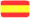SPANISCH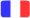FRANZÖSISCH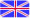ENGLISCH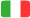ITALIENISCH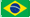PORTUGIESISCH